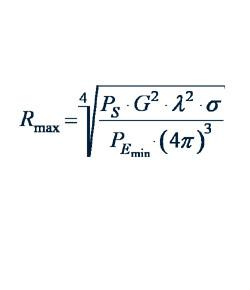# Steps Of Balancing Equations Quiz

9 Questions | Attempts: 1769SettingsA simple way to learn: practicing!  By applying the Law of conservation of mass it is easy to have any chemical equation balanced!  A few rules and everything is possible!

• 1.
What happens in a chemical reaction?
• A.

Atoms are destroyed

• B.

Atoms are created

• C.

Atoms are rearranged

• D.

Atoms are heated or cooled

• 2.
a ssa     A substance that undergoes a change in a chemical reaction is
• A.

A product

• B.

A chemical

• C.

A reactant

• D.

An enzyme

• 3.
A chemical equation is balanced by changing or adding
• A.

Chemical symbols

• B.

Subscripts

• C.

Coefficients

• D.

Reactants

• 4.
A balanced chemical equation shows the proportions of reactants and products necessary for
• A.

The reaction to occur

• B.

Mass to be conserved.

• C.

Energy use to be minimized.

• D.

Electrolysis to occur.

• 5.
If you start with 5 mol of O2 in the reaction 2Mg + O2 ----> 2MgO, how many moles of Mg will you need?
• A.

4

• B.

5

• C.

8

• D.

10

• 6.
What coefficients will satisfy the following equation Hg  +  O2  ---->  HgO
• A.

1,1,1

• B.

2,2,1

• C.

2,1,2

• D.

1,2,2

• 7.
What coefficients will satisfy the following equation AgNO3  +  NaCl  ---->  AgCl   +   NaNO
• A.

2,2,2,1

• B.

1,1,1,1

• C.

1,2,1,2

• D.

2,1,2,1

• 8.
What coefficients will satisfy the following equation Al  + O2  --> Al2O3
• A.

1,3,2

• B.

4,3,2

• C.

2,2,2

• D.

2,1,3

• 9.
What coefficients will satisfy the following equation H2 + O2 --->  H2O
• A.

2,2,1

• B.

2,1,2

• C.

4,1,2

• D.

2,2,2

## Related TopicsBack to top
×

Wait!
Here's an interesting quiz for you.